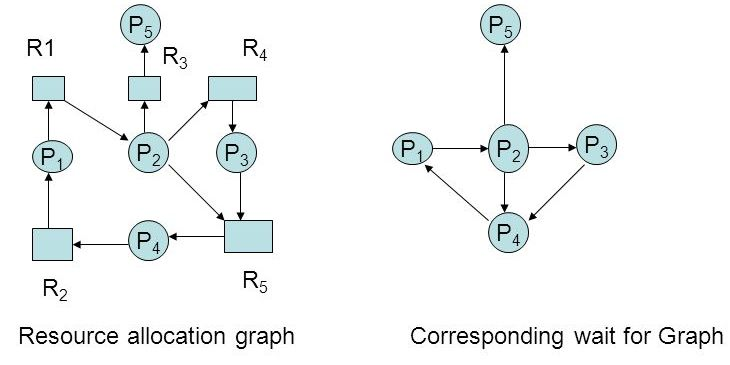• In my previous post, I had explained Banker’s algorithm as a “Deadlock Avoidance” technique.  We discussed basic concept of bankers’ algorithm as well as features and limitations of it.
• In this post, I am going to discuss Deadlock Detection techniques that deals with how deadlock in the system can be detected by using different techniques and methods.
• If a system does not implement a deadlock-prevention or a deadlock avoidance algorithm then system may have deadlock.
• In this situation the system may use:
1. Algorithm to check the stat of the system for possible deadlock detection.
2. An algorithm to recover from the deadlock
• Deadlock detection and recovery scheme includes run-time costs of maintaining and executing detection algorithm as well as possible loss of data from recovery.
• The algorithm should be capable of detecting deadlock for both:
1. Single instance of each resource type and
2. Several instance of each resource type.
1. ### Single Instance of each Resource Type

• If all resources have only single instance, we can use one of the form of the resource allocation graph called a wait for graph to detect deadlock
• We can prepare this graph from the resource-allocation graph by removing the resource nodes and collapsing the appropriate edges.
• A deadlock exists in the system if and only if the wait-for graph contains cycle.
• An algorithm to detect a cycle in a graph requires an order of n^2 operations, where n is the number of vertices in the graph.
• the following diagram represents resource allocation graph as well as its corresponding wait-for-graph.1. ### Several Instance of each Resource Type

• The wait-for graph scheme is not applicable in a system with several instance of each resource type.
• We have to use deadlock detection algorithms that can be used for such system.
• Such algorithm uses some time-variant data structures similar to those used in banker’s algorithm.
1. Request: An n X m matrix to represent the current request of each process.
2. Allocation: An n X m matrix to represent the number of resources of each type currently allocated to each process.
3. Available:  A vector of length m to represents the number of available resources of each type.
• Execution of deadlock detection algorithm depends on two criteria:
1. How many processes will be affected by the deadlock if deadlock occur?
2. How often deadlock is likely to occur?
• Execution of deadlock detection algorithm for every resource request will add extra overhead on the system.
• In my next post, I will explain Deadlock Recovery Scheme so please stay tuned!